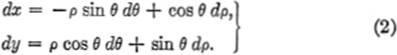﻿ ﻿LENGTH OF ARC - Curvature - The Calculus Primer

## The Calculus Primer (2011)

### Chapter 29. LENGTH OF ARC

8—1.Differential Arc Length. In order to understand the nature of curvature, it will be necessary to discuss what is meant by differential arc length. Let the curve in the figure represent the function y = f(x), and let s represent the length of the arc measured from a definite, initial point P0 to any point P(x,y) of the curve. Since the length s will depend upon the position of P0 on the curve, s is clearly a function of x. Thus suppose that as x takes on an increment Δx, the corresponding increment of s is Δs, and the corresponding point on the curve is R(x + Δx, y + Δy). Intuitively, from the figure, we note thatPR2 = (Δx)2 + (Ay)2,(1)

and henceAgain intuitively, we see that, as Δx approaches zero, arc Δs and chord PR become more and more nearly equal, so thatLet us now transform the left-hand member of (2) by multiplying both numerator and denominator by (Δs)2, and rewriting; thusIn other words, as s increases with x, we haveIn differential notation:The right-hand member of [1a] is an expression for the differential arc length ds.

It often happens that the given function is of the form x = ø(y), and s is an increasing function of y. In this case the corresponding formulas for differential arc length, by similar reasoning, are found to be8—2.Differential Arc Length for Equations in Parametric Form. If the function to be considered is given by a set of parametric equations, let us say

x = f(t), y = ø(t)

where t is the parameter, we may proceed as follows.

From equation (5), §8—1,or, clearing of fractions,

(ds)2 = (dx)2 + (dy)2.(1)

Since x = f(t) and y = ø(t),

dx = f′(t) dt, and dy = ø′ (t) dt.(2)

Substituting (2) in (1):

(ds)2 = [f′(t) dt]2 + [ø′ (t) dt]2,8—3.Differential Arc Length in Polar Coordinates. If the function whose differential arc length is desired is expressed in polar coordinates, the corresponding formulas are easily derived by employing the usual transformation formulas from rectangular to polar coordinates, namely,

x = ρ cos θ, y = ρ sin θ,(1)

Differentiating (1), using differential notation:Substituting in , §19—2:

(ds)2 = (−ρ sin θ dθ + cos θ dρ)2 + (ρ cos θ dθ + sin θ dp)2.

Simplifying:

(ds)2 = ρ2 (sin2 θ + cos2 θ)()2 + (sin2 θ + cos2 θ) (dp)2,

(ds)2 = ρ2()2 + (dp)2.[3a]To sum up, the differential length of arc is given by the formulas:Thus the differentials dx, dy, and ds form the sides of a right triangle in which

(ds)2 = (dx)2 + (dy)2.

Similarly, the differentials dρ, ρ dθ, and ds are related in such a way that they represent a right triangle whose hypotenuse is ds, and whose sides are and ρ dθ; or

(ds)2= (dp)2+(ρdθ)2.

﻿